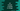# C# program to convert a double to integer## C# program to convert a double to integer:

In this post, we will learn how to convert a double to integer in C#. .NET provides a class called Convert which can be used to convert a base data type to another data type.

We will use Convert to convert a double to integer. Convert provides the method ToInt32 which can be used for double to integer conversion.

In this post, I will show you how to use ToInt32 and its definition.

## ToInt32 method definition:

Actually ToInt32 method can take different types of parameters. But, in this example, we will use the below variant:

``public static int ToInt32(Double v);``

It is a static method which takes one double value as the parameter. v is the double-precision floating point number. It returns one signed 32-bit integer.

If v is between two whole numbers, it returns the even number. For example, 2.5 is converted to 2 and 9.5 is converted to 10.

### Exception:

ToInt32 may throw OverflowException if v is greater than MaxValue or less than MinValue.

## Example program:

Let’s try ToInt32 with one program:

``````using System;

public class Program
{
public static void Main()
{
double[] arr = new double[] { -10, -10.5, -10.1, -9.5, 11, 12, 13.1, 13.4, 13.5, 13.6, 14.1, 14.4, 14.5, 14.6 };

foreach (double i in arr){
Console.WriteLine(i+" : "+Convert.ToInt32(i));
}
}
}``````

In this program, we have created one double array with different double values arr and we are converting each doubles in the array using a foreach loop.

If you run this program, it will give the below output:

``````-10 : -10
-10.5 : -10
-10.1 : -10
-9.5 : -10
11 : 11
12 : 12
13.1 : 13
13.4 : 13
13.5 : 14
13.6 : 14
14.1 : 14
14.4 : 14
14.5 : 14
14.6 : 15``````

### Example to take a double value as input and convert it to integer:

Let’s try another program. In this program, we are taking one double value as input from the user and converting it to integer using Convert.ToInt32.

``````using System;

public class Program
{
public static void Main()
{
double d;
int i;

Console.WriteLine("Enter a double value: ");
i = Convert.ToInt32(d);

Console.WriteLine(d+" : "+i);
}
}``````

Here,

• we have created one double variable d and one integer variable i.
• It reads the user entered value using Console.ReadLine and converted that value to double using double.Parse method.
• This double value is again converted to integer by using Convert.ToInt32 and it is stored in i.
• The last line is printing both double value and its converted integer value.

If you run this program, it will print output as like below:

``````Enter a double value:
12.3
12.3 : 12

Enter a double value:
13.5
13.5 : 14``````